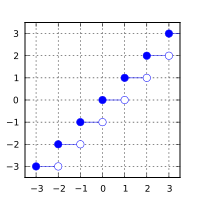/C++

# std::floor, std::floorf, std::floorl

Defined in header `<cmath>`
(1)
```float       floor ( float num );
double      floor ( double num );
long double floor ( long double num );```
(until C++23)
```constexpr /* floating-point-type */
floor ( /* floating-point-type */ num );```
(since C++23)
`float       floorf( float num );`
(2) (since C++11)
(constexpr since C++23)
`long double floorl( long double num );`
(3) (since C++11)
(constexpr since C++23)
Defined in header `<cmath>`
```template< class Integer >
double      floor ( Integer num );```
(A) (constexpr since C++23)
1-3) Computes the largest integer value not greater than `num`. The library provides overloads of `std::floor` for all cv-unqualified floating-point types as the type of the parameter. (since C++23)
 `double` (since C++11)

### Parameters

 num - floating-point or integer value

### Return value

If no errors occur, the largest integer value not greater than `num`, that is ⌊num⌋, is returned.

Return value`num`

### Error handling

Errors are reported as specified in `math_errhandling`.

If the implementation supports IEEE floating-point arithmetic (IEC 60559),

• The current rounding mode has no effect.
• If `num` is ±∞, it is returned, unmodified
• If `num` is ±0, it is returned, unmodified
• If `num` is NaN, NaN is returned

`FE_INEXACT` may be (but isn't required to be) raised when rounding a non-integer finite value.

The largest representable floating-point values are exact integers in all standard floating-point formats, so this function never overflows on its own; however the result may overflow any integer type (including `std::intmax_t`), when stored in an integer variable.

The additional overloads are not required to be provided exactly as (A). They only need to be sufficient to ensure that for their argument `num` of integer type, `std::floor(num)` has the same effect as `std::floor(static_cast<double>(num))`.

### Example

```#include <cmath>
#include <iostream>

int main()
{
std::cout << std::fixed
<< "floor(+2.7) = " << std::floor(+2.7) << '\n'
<< "floor(-2.7) = " << std::floor(-2.7) << '\n'
<< "floor(-0.0) = " << std::floor(-0.0) << '\n'
<< "floor(-Inf) = " << std::floor(-INFINITY) << '\n';
}```

Output:

```floor(+2.7) = 2.000000
floor(-2.7) = -3.000000
floor(-0.0) = -0.000000
floor(-Inf) = -inf```

 ceilceilfceill (C++11)(C++11) nearest integer not less than the given value (function) trunctruncftruncl (C++11)(C++11)(C++11) nearest integer not greater in magnitude than the given value (function) roundroundfroundllroundlroundflroundlllroundllroundfllroundl (C++11)(C++11)(C++11)(C++11)(C++11)(C++11)(C++11)(C++11)(C++11) nearest integer, rounding away from zero in halfway cases (function) C documentation for `floor`